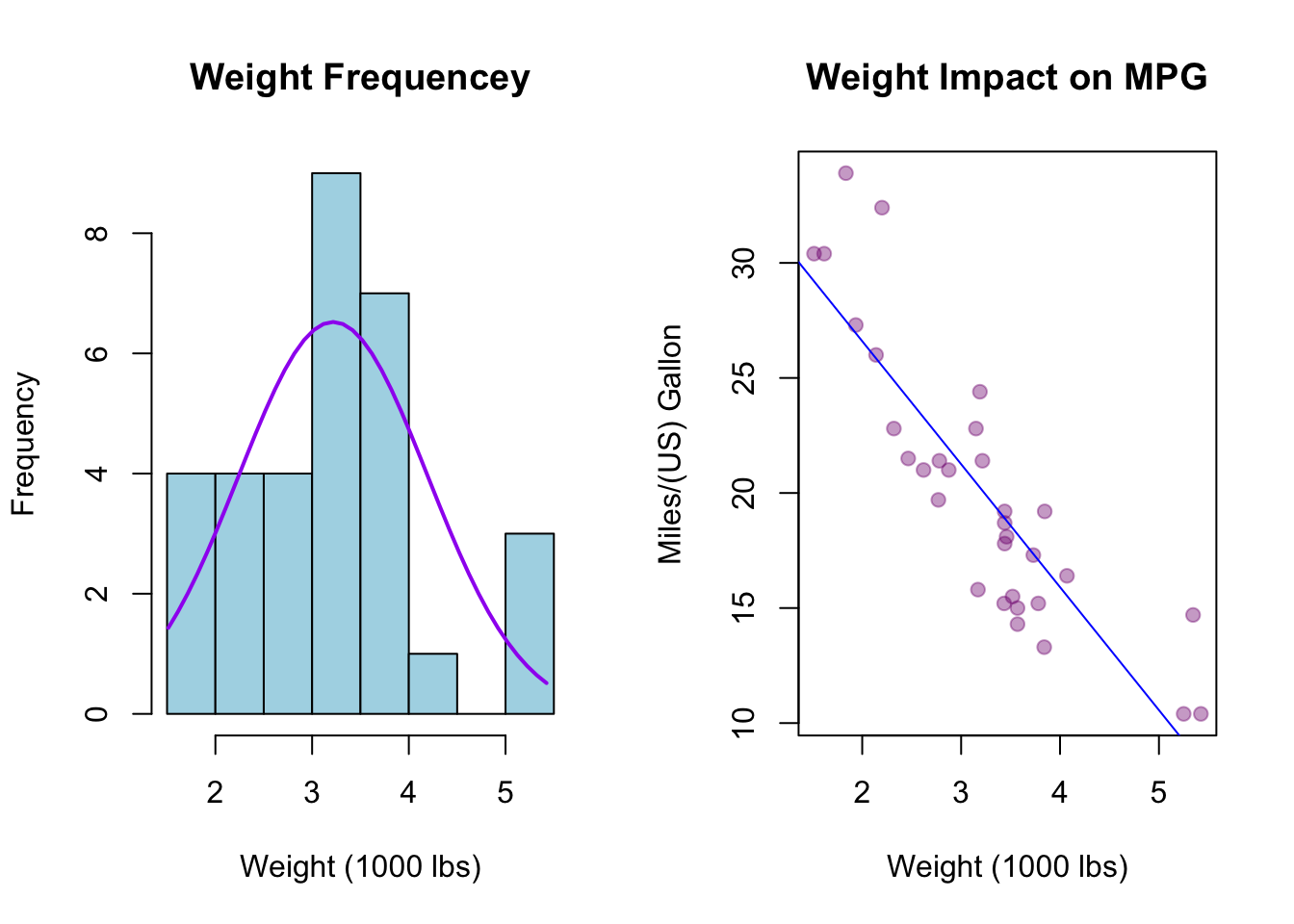#View Both Plots Side By Side
par(mfrow = c(1,2))

#Weight Frequency Histogram
#=======================================================
#Set X Value
xval <- mtcars$wt #Draw Histogram h <- hist(xval, breaks = 12, col = "lightblue", xlab = "Weight (1000 lbs)", main = "Weight Frequencey") #Draw Normal Fit Line xfit <- seq(min(xval),max(xval),length=40) yfit<-dnorm(xfit,mean=mean(xval),sd=sd(xval)) yfit <- yfit*diff(h$mids[1:2])*length(xval)
lines(xfit, yfit, col="purple", lwd=2)

#Weight Impact on MPG Plot
#=======================================================
#Set Axis Values
xval <- mtcars$wt yval <- mtcars$mpg

abline(regression, col = "blue")